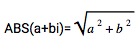IMABS

Returns the absolute value of a complex number.

IMABS("3+4i")

IMABS("6j")

Syntax

IMABS(number)

• number - The complex number to calculate the absolute value of.

Notes

• The absolute value (or modulus) is defined as follows:
•